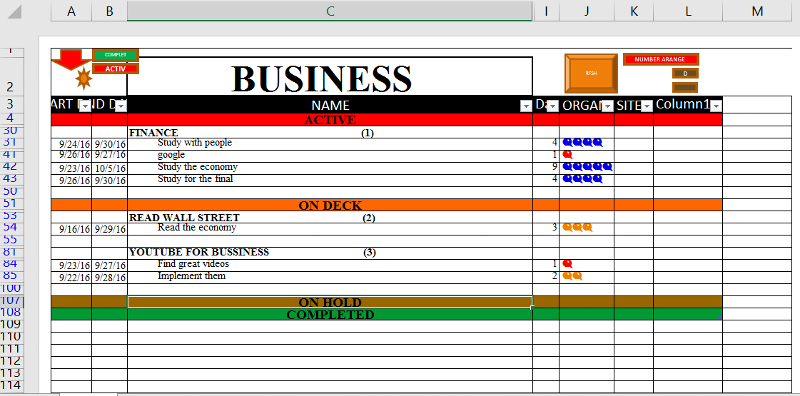# Changing amount of letter and color on column "J" when numbers on are being changed in column "I" automatically.

Hello guys i am trying to find out if this is possible, thanks again for your time and help.

On the "J" column have the Letter (Q) display no more than five times with the color of BLUE if on column "I" there is a number gretter than 5
On the "J" column have the Letter (Q) display Color orange if number is three on the "I" column with numbers of (Q) being shown equal to those of "I" column
On the "J" column have the Letter (Q) display Color Red if "I" column display onecompleted-9-26-OpEN.xlsm
###### Who is Participating?
I wear a lot of hats...

"The solutions and answers provided on Experts Exchange have been extremely helpful to me over the last few years. I wear a lot of hats - Developer, Database Administrator, Help Desk, etc., so I know a lot of things but not a lot about one thing. Experts Exchange gives me answers from people who do know a lot about one thing, in a easy to use platform." -Todd S.

IT ManagerCommented:
Yes, it's possible with VBA (and even trigger it on column I edits).
I'll have code later (unless someone beats me), since I'm not free at the moment.
Author Commented:
Thanks for your response Kimputer, forgot to mention to have the Q letters in (BOLD) WITH (SNAP ITC FONT)
IT ManagerCommented:
The following code should be on the same sheet as where you want the changes to happen:

``````Private Sub Worksheet_Change(ByVal Target As Range)

If Target.Column = 9 Then
If Target.Value > 0 Then
If Target.Value = 1 Then
Cells(Target.Row, 10).Value = "Q"
Cells(Target.Row, 10).Font.Color = -16776961
ElseIf Target.Value = 2 Then
Cells(Target.Row, 10).Value = "QQ"
Cells(Target.Row, 10).Font.ThemeColor = xlThemeColorAccent2
ElseIf Target.Value = 3 Then
Cells(Target.Row, 10).Value = "QQQ"
Cells(Target.Row, 10).Font.ThemeColor = xlThemeColorAccent2
ElseIf Target.Value = 4 Then
Cells(Target.Row, 10).Value = "QQQQ"
Cells(Target.Row, 10).Font.ThemeColor = xlThemeColorAccent2
ElseIf Target.Value > 4 Then
Cells(Target.Row, 10).Value = "QQQQQ"
Cells(Target.Row, 10).Font.Color = -65536
End If
Cells(Target.Row, 10).Font.Name = "Snap ITC"
Cells(Target.Row, 10).Font.FontStyle = "Bold"
Cells(Target.Row, 10).Font.Size = 8
End If
End If

End Sub
``````
Author Commented:
Hello there Kimputer, the column where i have it programmed has no module or sheet that i know of i would attach the file here, so that it may be easier for you to suggest another way to have it work.
completed-9-24-OPEN-1.xlsm
IT ManagerCommented:
Open Excel file, press ALT+F11, navigate to Sheet3(GENERAL), copy & paste code there. Here's some updated code, as well as the excel file attached if you really can't do it:

``````Private Sub Worksheet_Change(ByVal Target As Range)

If Target.Column = 9 Then
If Target.Value > 0 Then
If Target.Value = 1 Then
Cells(Target.Row, 10).Value = "Q"
Cells(Target.Row, 10).Font.Color = -16776961
ElseIf Target.Value = 2 Then
Cells(Target.Row, 10).Value = "QQ"
Cells(Target.Row, 10).Font.Color = 26367
ElseIf Target.Value = 3 Then
Cells(Target.Row, 10).Value = "QQQ"
Cells(Target.Row, 10).Font.Color = 26367
ElseIf Target.Value = 4 Then
Cells(Target.Row, 10).Value = "QQQQ"
Cells(Target.Row, 10).Font.Color = 26367
ElseIf Target.Value > 4 Then
Cells(Target.Row, 10).Value = "QQQQQ"
Cells(Target.Row, 10).Font.Color = -65536
End If
Cells(Target.Row, 10).Font.Name = "Snap ITC"
Cells(Target.Row, 10).Font.FontStyle = "Bold"
Cells(Target.Row, 10).Font.Size = 8
End If
If Target.Value = "" Then
Cells(Target.Row, 10).Value = ""
End If
End If

End Sub
``````
completed-9-24-OPEN-1.xlsm
Author Commented:
Thanks for your response, i see now what was the issue. It works great, but i forgot to mention that the "I"column has a code that goes like this (=IF(B6<>"",B6-TODAY(),"")).

***Any way we can make it work with this code or if you have a better suggestion please let me know so that i may have the "I" column calculating the present day and how many days would be left when calculating it with the "B" column.
***also with the "J" column to have the (Q letter) representing the amount of days left.
IT ManagerCommented:
It's still possible, triggers will be ONLY column 2 now.

``````Private Sub Worksheet_Change(ByVal Target As Range)
Dim eval As Long
If Target.Column = 2 Then
eval = Cells(Target.Row, 2).Value - Date
If eval > 0 Then
If eval = 1 Then
Cells(Target.Row, 10).Value = "Q"
Cells(Target.Row, 10).Font.Color = -16776961
ElseIf eval = 2 Then
Cells(Target.Row, 10).Value = "QQ"
Cells(Target.Row, 10).Font.Color = 26367
ElseIf eval = 3 Then
Cells(Target.Row, 10).Value = "QQQ"
Cells(Target.Row, 10).Font.Color = 26367
ElseIf eval = 4 Then
Cells(Target.Row, 10).Value = "QQQQ"
Cells(Target.Row, 10).Font.Color = 26367
ElseIf eval > 4 Then
Cells(Target.Row, 10).Value = "QQQQQ"
Cells(Target.Row, 10).Font.Color = -65536
End If
Cells(Target.Row, 10).Font.Name = "Snap ITC"
Cells(Target.Row, 10).Font.FontStyle = "Bold"
Cells(Target.Row, 10).Font.Size = 8
Else
Cells(Target.Row, 10).Value = ""
End If
If Cells(Target.Row, 2) = Empty Then
Cells(Target.Row, 10).Value = ""
End If
End If

End Sub
``````

Since code is now dependent on other input than yourself, need to know default action if value is < 0
(For now, it's just emptying the cell).

Experts Exchange Solution brought to you by